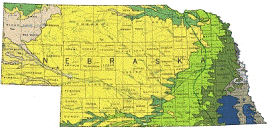## US Geological Survey## USGS Staff -- Published Research

#### Date of this Version

2001

Published in JOURNAL OF GEOPHYSICALR ESEARCH, VOL. 106, NO. B12, PAGES 30, 701-30, 713, DECEMBER 10, 2001

#### Abstract

We determine the scaling relationships between earthquake stress drop and recurrence interval t, that are implied by laboratory-measured fault strength. We assume that repeating earthquakes can be simulated by stick-slip sliding using a spring and slider block model. Simulations with static/kinetic strength, time-dependent strength, and rate- and state-variable dependent strength indicate that the relationship between loading velociety and recurrence intercal can be adequately described be the power law VL ∞ tnr, where n -1. Deviations from n -1 arise from second order effects on strength, with n> -1 corresponding to apparent time-dependent strengthening and n< -1 corresponding to weakening. Simulations with rate and state-variable equations show that dynamic shear stress drop Δ τd scales with recurrence as dΔτd/dlntr ≤ σe(b-a), where σe is the effective normal stress, μ=τ/σe, and (a-b)=dμ38/dlnV is the steady-state slip rate dependence of strength. In addition, accounting for seismic energy radiation, we suggest that the static shear stress drop Δτs scales as dΔτs/dlntr ≤σe(1+ ∫)(b-a), where ∫ is the fractional overshoot. The variation of Δτs with lntr for earthquake stress drops is somewhat larger than implied by room temperature laboratory values of ∫ and b-a. However, the uncertainty associated witht the seismic data is large and the discrepancy between the seismic observations and the rate of strengthening predicted by room temperature experiments is less than an order of magnitude.

COinS